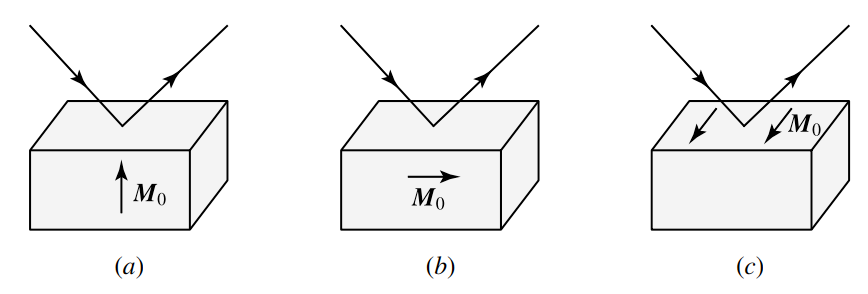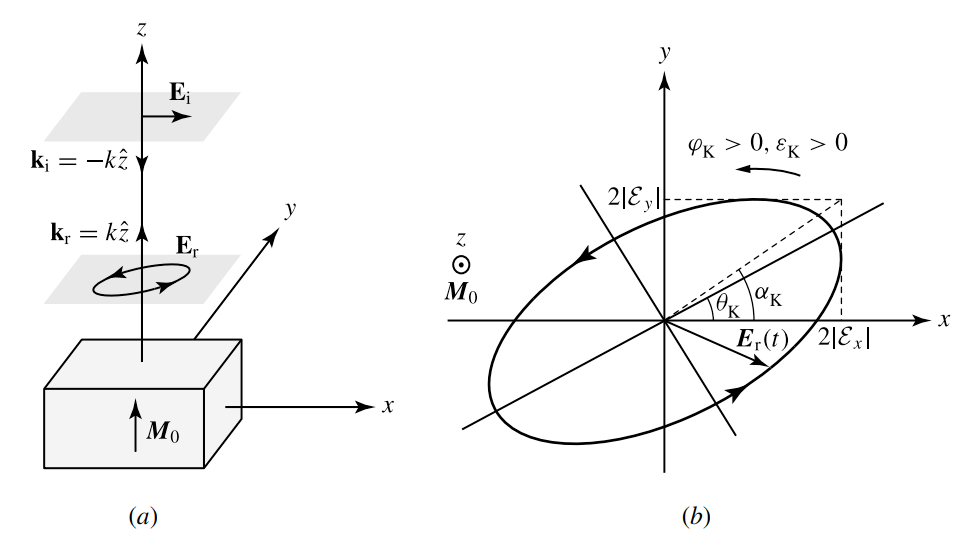# Magneto-optic Kerr effect

This is a continuation from the previous tutorial - Faraday effect.

Reflection of a polarized optical wave from the surface of a material with an internal magnetization or from that of one subject to an external magnetic field results in a change of the polarization state and/or the reflectivity that is dependent on the magnetization or the magnetic field. This phenomenon is known as the magneto-optic Kerr effect.

It is totally unrelated to, and should not be confused with, the electro-optic Kerr effect discussed in the electro-optic effect tutorial. The only connection between the two is that both were discovered by J. C. Kerr.

The magneto-optic Kerr effect stems from the same physical origin as the Faraday effect. Both are first-order magneto-optic effects. The distinction between the two is that the Faraday effect is associated with light in transmission whereas the Kerr effect is associated with light in reflection.

The Kerr effect can be observed from the surface of a material that has no internal magnetization but is subject to an external magnetic field or from the surface of a ferromagnetic or ferrimagnetic material. The latter is more important than the former in practical applications, and we shall take it to be the case in the following discussions. The general concepts and the results obtained can be applied to the former case in a similar fashion.

There are three configurations, shown in figure 7-2 below, of the magneto-optic Kerr effect.Figure 7-2. Three configurations of magneto-optic Kerr effect: (a) polar Kerr effect, (b) longitudinal Kerr effect, and (c) transverse Kerr effect.

In the polar Kerr effect, the magnetization $$\pmb{M}_0$$ is normal to the surface of the magnetic medium from which the optical wave is reflected.

In the longitudinal Kerr effect, also known as the meridional Kerr effect, $$\pmb{M}_0$$ is parallel to the surface of the medium and lies in the plane of incidence.

In the transverse Kerr effect, also known as the equatorial Kerr effect, $$\pmb{M}_0$$ is normal to the plane of incidence.

Consider the simple and most common situation in which the incident optical wave traveling in an isotropic, nonmagnetic medium is reflected from the surface of an isotropic magnetic medium. The TE and TM polarizations are both normal modes for the incident and the reflected optical waves traveling in the isotropic, nonmagnetic medium.

However, they are not normal modes for the wave traveling in the magnetic medium because of circular birefringence of the medium. Consequently, upon reflection from the magnetic surface, the TE-polarized component of an incident wave can be coupled to the TM-polarized component, and vice versa.

All of the three Kerr effects can then be generally expressed in terms of a tensor of reflection coefficients:

$\tag{7-31}\begin{bmatrix}E_s^r\\E_p^r\end{bmatrix}=\begin{bmatrix}r_{ss}&r_{sp}\\r_{ps}&r_{pp}\end{bmatrix}\begin{bmatrix}E_s^i\\E_p^i\end{bmatrix}$

where the subscripts $$s$$ and $$p$$ represent TE and TM polarizations, for s and p waves, respectively, and the superscripts $$i$$ and $$r$$ indicate incident and reflected fields, respectively.

From the discussions in the magneto-optic effects tutorial and the Faraday effects tutorial, we know that only the electric field components that are perpendicular to the magnetization interact with the magnetization and see the circular birefringence.

For polar and longitudinal Kerr effects, both TE and TM polarizations interact with $$\pmb{M}_0$$ because both have nonvanishing components perpendicular to $$\pmb{M}_0$$. This interaction results in nonvanishing $$r_{sp}$$ and $$r_{ps}$$ due to coupling between the TE- and TM-polarized fields through circular birefringence of the magnetic medium.

The values of $$r_{sp}$$ and $$r_{ps}$$ are proportional to $$\xi$$. Their phases are different from those of $$r_{ss}$$ and $$r_{pp}$$. Consequently, a linearly polarized incident wave is turned into an elliptically polarized wave with the direction of its major axis rotated away from the initial plane of polarization.

Because $$r_{sp}$$ and $$r_{ps}$$ are linearly dependent on $$\pmb{M}_0$$, they change sign when the direction of $$\pmb{M}_0$$ is reversed. Consequently, both the angle of rotation and the ellipticity of the elliptically polarized reflected wave change sign upon reversing the direction of $$\pmb{M}_0$$

For the transverse Kerr effect, only TM polarization interacts with $$\pmb{M}_0$$ because the electric field of the TE polarization is parallel to $$\pmb{M}_0$$. Then, $$r_{sp}=r_{ps}=0$$, and $$r_{ss}$$ is independent of $$\pmb{M}_0$$. The net effect is only a magnetically induced change in $$r_{pp}$$. This change is linearly proportional to $$\xi$$. It also changes sign when the direction of $$\pmb{M}_0$$ is reversed.

The Kerr effect in each configuration varies with the angle of incidence. In a majority of cases, particularly in those with small incident angles, the polar configuration haws a larger effect than both longitudinal and transverse configurations.

The Kerr effect in the polar configuration increases as the angle of incidence decreases. At normal incidence, the Kerr effect reaches its maximum in the polar configuration, but it vanishes in both longitudinal and transverse configurations.

Therefore, among the three different configurations in combination with all possible angles of incidence, the polar configuration at normal incidence has the most pronounced Kerr effect and is most useful for practical applications such as magneto-optic recording.

It is also the simplest to analyze. In the following, we consider this specific case.

We consider the polar Kerr effect at normal incidence from free space on the surface of a ferromagnet or ferrimagnet. The magnetic material is assumed to be isotropic or uniaxial with its magnetization parallel to the optical axis along the $$z$$ direction. The incident wave propagates in the negative $$z$$ direction while the reflected wave propagates in the positive $$z$$ direction, as shown in figure 7-3(a) below.Figure 7-3. Polar Kerr effect at normal incidence: (a) configuration showing the designation of the coordinates and the direction of the magnetization and (b) sense of Kerr rotation and that of Kerr ellipticity with respect to the direction of magnetization for positive values of $$\theta_\text{K}$$ and $$\epsilon_\text{K}$$.

Without loss of generality in the case under consideration, a linearly polarized incident beam can be assumed to be polarized in the $$x$$ direction:

$\tag{7-32}\mathbf{E}_\text{i}(z,t)=\hat{x}\mathcal{E}\text{e}^{-\text{i}kz-\text{i}\omega{t}}=\frac{\mathcal{E}}{\sqrt2}(\hat{e}_++\hat{e}_-)\text{e}^{-\text{i}kz-\text{i}\omega{t}}$

In free space, $$\hat{e}_+$$ and $$\hat{e}_-$$ are also normal modes of propagation like $$\hat{x}$$, all of which have the same propagation constant $$k$$. In addition, $$\mathcal{E}_+=\mathcal{E}_-=\mathcal{E}/\sqrt2$$.

In the magnetic material, $$\hat{x}$$ is not a normal mode any more, and the normal modes $$\hat{e}_+$$ and $$\hat{e}_-$$ have different propagation constants.

As discussed in the Faraday effect tutorial, when the direction of $$\pmb{M}_0$$ is fixed, the values of the propagation constants $$k^+$$ and $$k^-$$, as well as those of the principal indices of refraction $$n_+$$ and $$n_-$$, for the normal modes $$\hat{e}_+$$ and $$\hat{e}_-$$, respectively, are independent of whether the wave propagates in a parallel or antiparallel direction with respect to the direction of $$\pmb{M}_0$$. Only the handedness of $$\hat{e}_+$$ and $$\hat{e}_-$$ is interchanged when the propagation direction is reversed.

As independent eigenmodes with characteristic indices of refraction, the circularly polarized modes do not couple to each other on reflection. They have the following clearly defined reflection coefficients:

$\tag{7-33}r_+=\frac{1-n_+}{1+n_+},\qquad{r_-=\frac{1-n_-}{1+n_-}}$

for the $$\hat{e}_+$$ and $$\hat{e}_-$$ modes, respectively.

According to the configuration defined in figure 7-3 above, the reflected wave propagates in the positive $$z$$ direction. The wave reflected back from the magnetic surface back into free space, where $$\hat{e}_+$$ and $$\hat{e}_-$$ modes have the same propagation constant $$k$$, is then described by

\tag{7-34}\begin{align}\mathbf{E}_r(z,t)&=\hat{e}_+r_+\mathcal{E}_+\text{e}^{\text{i}kz-\text{i}\omega{t}}+\hat{e}_-r_-\mathcal{E}_-\text{e}^{\text{i}kz-\text{i}\omega{t}}\\&=\frac{\mathcal{E}}{\sqrt2}(\hat{e}_+r_++\hat{e}_-r_-)\text{e}^{\text{i}kz-\text{i}\omega{t}}\\&=\frac{\mathcal{E}}{2}[\hat{x}(r_++r_-)+\text{i}\hat{y}(r_+-r_-)]\text{e}^{\text{i}kz-\text{i}\omega{t}}\end{align}

The $$x$$ and $$y$$ components of this reflected field are $$\mathcal{E}_x^r=\mathcal{E}(r_++r_-)/2$$ and $$\mathcal{E}_y^r=\text{i}\mathcal{E}(r_+-r_-)/2$$, respectively. Clearly, the reflected wave is elliptically polarized.

As described in the polarization of light tutorial, the characteristics of an elliptically polarized field can be completely specified by either of two sets of parameters: ($$\alpha,\varphi$$) and ($$\theta,\epsilon$$). Using (62) [refer to the polarization of light tutorial], the parameters $$\alpha_\text{K}$$ and $$\varphi_\text{K}$$ that characterize the elliptically polarized field in (7-34) are given by

$\tag{7-35}\tan\alpha_\text{K}\text{e}^{\text{i}\varphi_\text{K}}=\frac{\mathcal{E}_y^r}{\mathcal{E}_x^r}=\text{i}\frac{r_+-r_-}{r_++r_-}$

where the subscript $$\text{K}$$ for the parameters indicates their association with the Kerr effect.

Because it is generally true in practical magnetic materials that $$|r_+-r_-|\ll|r_++r_-|$$, the value of $$\alpha_\text{K}$$ is very small. So are the values of the corresponding $$\theta_\text{K}$$ and $$\epsilon_\text{K}$$ parameters.

Using the relations in (66) and (67) [refer to the polarization of light tutorial] in the limit of small values for these parameters, we have

$\tag{7-36}\tan\alpha_\text{K}\text{e}^{\text{i}\varphi_\text{K}}\approx\alpha_\text{K}\text{e}^{\text{i}\varphi_\text{K}}\approx\theta_\text{K}+\text{i}\epsilon_\text{K}$

Combining (7-35) and (7-36), we find

$\tag{7-37}\theta_\text{K}+\text{i}\epsilon_\text{K}=\text{i}\frac{r_+-r_-}{r_++r_-}=\text{i}\frac{n_+-n_-}{n_+n_--1}\approx-\text{i}\frac{\xi}{n_\perp(n_\perp^2-1)}$

Therefore, the Kerr rotation angle, $$\theta_\text{K}$$, and the Kerr ellipticity, $$\epsilon_\text{K}$$, are given by

$\tag{7-38}\theta_\text{K}=\text{Im}\left[\frac{\xi}{n_\perp(n_\perp^2-1)}\right],\qquad\epsilon_\text{K}=-\text{Re}\left[\frac{\xi}{n_\perp(n_\perp^2-1)}\right]$

respectively.

In case optical loss of nonmagnetic origin is small in the magnetic medium so that $$|n_\perp''|\ll{n_\perp'}$$, $$\theta_\text{K}$$ is caused solely by magnetic circular dichroism and is completely determined by $$\xi''$$ while $$\epsilon_\text{K}$$ is caused by magnetic circular birefringence and is determined by $$\xi'$$.

Note the distinction between the Faraday effect and the Kerr effect. Magnetic circular birefringence is the cause of Faraday rotation and Kerr ellipticity, whereas magnetic circular dichroism is the mechanism behind Kerr rotation and Faraday ellipticity.

As can be seen from (7-38), both $$\theta_\text{K}$$ and $$\epsilon_\text{K}$$ are linearly dependent on magnetization. Both depend strongly on the optical wavelength. They are intrinsic properties of a magnetic material that are generally determined by direct measurement.

Their values are small, normally on the order of $$\pm1^\circ$$ or less for most materials. Their signs are defined in a manner similar to how the sign of Faraday rotation is defined: $$\theta_\text{K}$$ is positive if the major axis of the ellipse describing the polarization of the reflected wave is rotated away from the direction of linear polarization of the incident wave in a sense that can be described by an axial vector pointing in the direction of $$\pmb{M}_0$$, and $$\epsilon_\text{K}$$ is positive if the elliptically polarized field at a fixed point in space rotates in the same sense as the electric current that can be associated with $$\pmb{M}_0$$. This convention is illustrated in figure 7-3(b) above.

Example 7-2

At 546 nm wavelength, iron at room temperature has a complex index of refraction of $$n=2.73+\text{i}3.3$$ and, when fully magnetized, a complex linear magneto-optic constant of $$\xi=-0.18+\text{i}0.74$$. A linearly polarized optical wave at 546 nm is normally incident on a fully magnetized iron surface in polar configuration, as shown in figure 7-3. Find the Kerr rotation angle and the Kerr ellipticity of the reflected wave.

Neglecting second-order magneto-optic effects, we can take $$n_\perp=n=2.73+\text{i}3.3$$. Then,

$\frac{\xi}{n_\perp(n_\perp^2-1)}=\frac{-0.18+\text{i}0.74}{(2.73+\text{i}3.3)[(2.73+\text{i}3.3)^2-1]}=0.0061-\text{i}0.0074$

Using (7-38), we find that $$\theta_\text{K}=-7.4\text{ mrad}\approx-0.42^\circ$$ and $$\epsilon_\text{K}=-6.1\text{ mrad}\approx-0.35^\circ$$. Because $$|\theta_\text{K}|\ll1$$ and $$|\epsilon_\text{K}|\ll1$$, the approximations made in (7-36) and (7-37) to obtain (7-38) are valid.

The next part continues with the optical isolators and circulators tutorial